GCSELevel 6-7AQAEdexcelOCRWJEC

A quadratic sequence is a sequence whose $n^{th}$ term formula is a quadratic i.e. it has an $n^2$ term, so takes the form,

$\textcolor{red}{a}n^2+\textcolor{blue}{b}n+\textcolor{limegreen}{c}$,

where $a, b,$ and $c$ are all numbers. The resulting sequences don’t have a common difference between each term as linear sequences do, but rather the difference between the differences remains the same.

Make sure you are happy with the following topics before continuing

Level 6-7GCSEAQAEdexcelOCRWJEC

## Finding the $n^{th}$ Term of a Quadratic Sequence ($a=1$)

Example: Find the $n^{th}$ term formula of the following quadratic sequence.

$2, 9, 18, 29, 42.$

Step 1: Find the difference between each term, and find the second differences (i.e. the differences between the differences);

To do this, we will first find the differences between the terms in the sequence.We can see that unlike a linear sequence, the differences aren’t the same. However, if we then look at the differences between those differences, we see the second differences are the same.

We now know the $n^{th}$ term formula is $\textcolor{red}{a}n^2+\textcolor{blue}{b}n+\textcolor{limegreen}{c}$.

To find the value of $\textcolor{red}{a}$ we find the second difference, which is $2$, and divide this by $2$. Hence $\textcolor{red}{a=1}$.

Step 2: Subtract $\textcolor{red}{a}n^2$ from the original sequence.

We still need to find $\textcolor{blue}{b}$ and $\textcolor{limegreen}{c}$. To do this we substitute the values of $n$ from $1$ to $5$ into $\textcolor{red}{a}n^2$, and write the results below the original sequence as shown.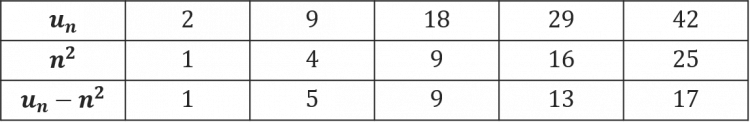$u_n =$ the original sequence.

If you look carefully at $u_n - n^2$ you will notice it is a linear sequence whose $n^{th}$ term will give us the missing letters of the quadratic $n^{th}$ term that we want.

Step 3: Find the $n^{th}$ term of the new linear sequence.The $n^{th}$ term for this linear sequence is $4n-3$.

Step 4: Write the final $n^{th}$ term for the full quadratic sequence.

This formula is precisely the last part of the quadratic $n^{th}$ term formula that we set out to find in the first place. Hence we get our final $n{th}$ term to be,

$n^2+4n-3$.

Level 6-7GCSEAQAEdexcelOCRWJEC
Level 6-7GCSEAQAEdexcelOCRWJEC

## Finding the $n^{th}$ Term of a Quadratic Sequence ($a\gt1$)

Example: Find the $n^{th}$ term formula of the following quadratic sequence.

$4, 15, 32, 55, 84$

Step 1: Find the difference between each term, and find the second differences (i.e. the differences between the differences);

We will first find the differences between the terms in the sequence.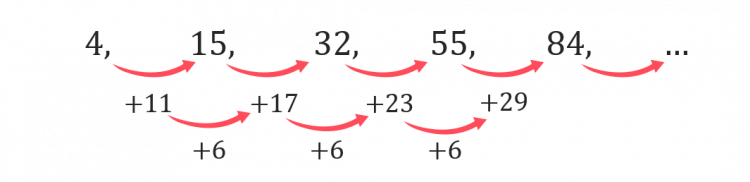To find the value of $a$ we find the second difference, which is $6$, and divide this by $2$. Hence $a=3$.

Step 2: Subtract $\textcolor{red}{3}n^2$

We still need to find $\textcolor{blue}{b}$ and $\textcolor{limegreen}{c}$. To do this we substitute the values of $3n^2$ from $1$ to $5$ into this, and write the results below the original sequence as shown.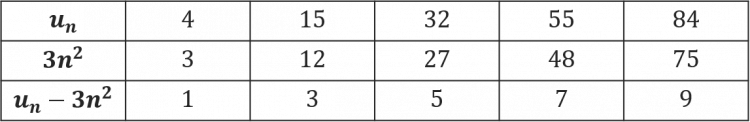$u_n =$ the original sequence

Here, we have subtracted our values for $3n^2$ from the actual sequence to form a linear sequence, the $n^{th}$ term of which will give us the missing letters of the quadratic $n^{th}$ term that we want.

Step 3: Find the $n^{th}$ term of the new sequence.The $n^{th}$ term for this linear sequence is $u_n=2n-1$.

Step 4: Write the final $n^{th}$ term for the full quadratic sequence.

This formula is precisely the last part of the quadratic $n^{th}$ term formula that we set out to find in the first place. Hence we get our final $n^{th}$ term to be,

$u_n=3n^2+2n-1$.

Level 6-7GCSEAQAEdexcelOCRWJEC

## Summary of Steps to find the $n^{th}$ Term

Every time you need to find the $n^{th}$ term formula for a quadratic sequence, the method is the same.

To find $\textcolor{red}{a}n^2+\textcolor{blue}{b}n+\textcolor{green}c$, the steps are:

1. Find the difference between each term, and find the second differences (i.e. the differences between the differences);
2. Check that the second differences are all the same, and then halve that number to get $\textcolor{red}{a}$;
3. Write out the terms of the sequence again, then substitute the first few values of $n$ into $an^2$ and write the results below the original sequence.
4. Subtract the elements of the second row from the elements above them in the first row.
5. Treat these differences like a sequence and find the $n^{th}$ term of that linear sequence.
6. Finally, combine the $\textcolor{red}{a}n^2$ that you found in step 2 with the $\textcolor{blue}{b}n+\textcolor{green}{c}$ that you found in step 5, and you’ve got your quadratic $n^{th}$ term formula.
Level 6-7GCSEAQAEdexcelOCRWJEC

## Note:

Subscript notation can be used to denote position to term and term to term rules.

For example

$x_{n+1}=x_n^2+5x-1$ shows that you square the previous term, add $5$ times the previous term and subtract $1$ to get the next term.

$y_n=4n^2-5n+3$ gives you a rule for the $n$th term of a sequence.

Level 6-7GCSEOCR

To generate the first $5$ terms of this sequence, we will substitute $n=1, 2, 3, 4, 5$ into the formula given.

\begin{aligned}n=1 \text{ gives } &(1)^2+6(1)-10=-3 \\n=2 \text{ gives } &(2)^2+6(2)-10=6 \\ n=3\text{ gives } &(3)^2+6(3)-10=17 \\ n=4 \text{ gives } &(4)^2+6(4)-10=30 \\ n=5 \text{ gives } &(5)^2+6(5)-10=45\end{aligned}

Hence the first five terms of the sequence are,

$-3, 6, 17, 30, 45$Gold Standard Education

a) To generate the first $4$ terms of this sequence, we will substitute $n=1, 2, 3, 4$ into the formula given.

\begin{aligned}n=1 \text{ gives } &(1)^2-2 =-1 \\ n=2 \text{ gives } &(2)^2-2 =4 \\ n=3 \text{ gives } &(3)^2-2=7 \\ n=4 \text{ gives } &(4)^2-2=14 \end{aligned}

b) Every term in this sequence is generated when an integer value of $n$ is substituted into $n^2-2$

Hence if we set $287$ to equal $n^2-2$, we can determine its position in the sequence,

$n^2-2=287$

making $n$ the subject,

$n=\sqrt{287+2}=17$

Hence $287$ is the $17^{th}$ term in the sequence.Gold Standard Education

Every term in this sequence is generated when an integer value of $n$ is substituted into $(n-1)^2$

Thus if we set $49$ to equal $(n-1)^2$, we can determine its position in the sequence,

\begin{aligned}(n-1)^2&=49 \\ n-1 &=\pm\sqrt{49} \\ n&=1 +7 = 8\end{aligned}

Hence $49$ is the $8^{th}$ term in the sequence, as $n$ can only be positive integers.Gold Standard Education

So, the $n^{th}$ term formula will take the form $an^2+bn+c$ where $a, b,$ and $c$ are numbers to be determined.

Firstly, we have to find the differences between the terms in the sequences, and then find the difference between the differences. Doing so, we find,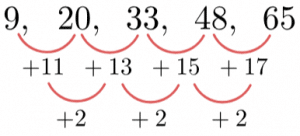The second difference is the same, as expected, therefore $a$ is half of the second difference and so $a=1$

Now we need to find $b$ and $c$ by comparing the values generated by a sequence  of $n^2$, to the original sequence.

\begin{aligned} u_n &= 9 , 20, 33, 48, 65 \\ n^2 &= 1, 4, 9, 16, 25 \\ u_n - n^2 &= 8, 16, 24, 32, 40\end{aligned}

The difference, is a linear sequence whose $n^{th}$ term formula is precisely $bn+c$, so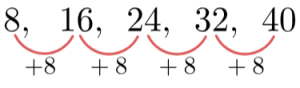The difference is $8$, so the $n^{th}$ term must be $8n+c$ where $c=0$

Therefore, we get the $n^{th}$ term formula of the quadratic to be,

$n^2+8n$Gold Standard Education

So, the $n^{th}$ term formula will take the form $an^2+bn+c$ where $a, b,$ and $c$ are numbers to be determined.

Firstly, we have to find the differences between the terms in the sequences, and then find the difference between the differences. Doing so, we find,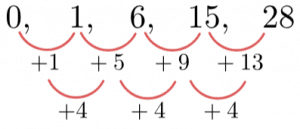The second difference is the same, as expected, therefore $a$ is half of the second difference and so $a=2$

Now we need to find $b$ and $c$ by comparing the values generated by a sequence  of $2n^2$, to the original sequence.

\begin{aligned}u_n & = 0, 1, 6, 15, 28 \\ 2n^2 & = 2, 8, 18, 32, 50 \\u_n-2n^2 & = -2, -7, -12, -17, -22\end{aligned}

The difference, is a linear sequence whose $n^{th}$ term formula is precisely $bn+c$, so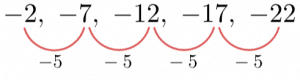The difference is $-5$, so the $n^{th}$ term must be $-5n+c$. Then, for $n=1$, we get $-5n=-5$, whereas the first term is not $-5$, but $-2$. To get from $-5$ to $-2$ we have to add $3$, so we must have that $c=3$, and thus the $n^{th}$ term is

$-5n+3$

Therefore, combining this with the first term in the quadratic that we found earlier, we get the $n^{th}$ term formula of the quadratic to be

$2n^2-5n+3$Gold Standard Education

## Quadratic Sequences Worksheet and Example Questions

### (NEW) Quadratic Sequences Exam Style Questions - MME

Level 6-7GCSENewOfficial MMEGold Standard Education

Level 4-5GCSEKS3